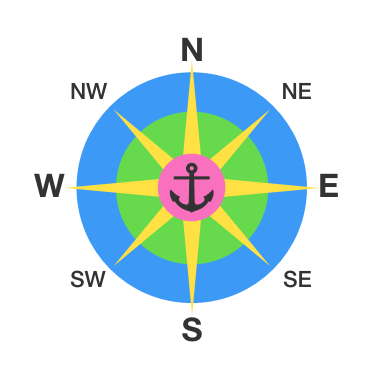# Find The Diamond!

Logic Level 3There is a diamond hidden on an $N\times N$ grid at location $(x_D,y_D)$, where $x_D$ and $y_D$ are integers.

Every guess, you suggest a pair of coordinates ($x_G$, $y_G$). And, if you get it wrong you are given a hint as to where to go to continue looking. You are told either NW, N, NE, E, SE, S, SW, or W:

• W implies $x_D < x_G$ and $y_D = y_G$
• NW implies $x_D < x_G$ and $y_D > y_G$
• etc.

If you can get the diamond with 10 guesses or less (i.e. at most 9 wrong guesses and one right one), you get to keep the diamond. What is the largest $N$ for which you can guarantee success?

×